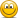CourseErrors Found in 2021 Study Materials P1.T2. Quantitative Methods

Nicole Seaman

Director of FRM Operations
Staff member
Subscriber
Please use this new thread to let David and I know about any errors, missing/broken links, etc. that you find in the 2021 materials that are published in the study planner under P1.T2. Quantitative Methods. This will keep our forum much more organized. We appreciate your cooperation!PLEASE NOTE: Our Practice Question sets already have links to their specific forum threads where you can post about any errors that you find. This thread is for any other materials (notes, spreadsheets, videos, etc.) where you might find errors.

Information needed for us to correct errors:

• Page number
• Error

dla00

New Member
Subscriber
Hello,

Regarding Chapter 1: Fundamentals of Probability, Study Notes.

On page 27 answer 300.1.C, the last term should be +0.75*6 or () before 6^2/16.

dla00

New Member
Subscriber
Hello,

Regarding Study Notes: Random Variables (Chapter 2).

A couple of questions:

1. On page 10, why do we have two f(x) for Bernoulli's pmf?
2. Just below the above, when defining CDF of Bernoulli, I think F(x)=1-p when 0 <= x < 1, e.g the last inequality should be strict (notes say x <= 1).

Thanks!

David Harper CFA FRM

David Harper CFA FRM
Staff member
Subscriber
Hi @dla00
1. Not sure why we have the second one: it's almost certainly that we retained a legacy expression from the prior reading. Although I don't like the second one, now that I look at it. You can see by the text that it's trying to operationalize the statement "The pmf is the difference of the CDFs evaluated at consecutive values in support of X" but I don't see the point of that in a Bernoulli. In a binomial it could be useful; i.e., binomial P(X = 5) = P(X ≤ 5) - P(X ≤ 4).
2. Yes, I agree, as does wikipedia https://en.wikipedia.org/wiki/Bernoulli_distribution . Tagged for fix. Thank you!

Garbanzo

Member
SubscriberP1.T2 Chapter 2 Random Variables, Study notes page 7:

should say:

"The cumulative DISTRIBUTION function (CDF) associates with either a pmf or PDF (i.e., the CDF can apply to either a continuous or DISCRETE random variable)."P1.T2 Chapter 2 Random Variables, Study notes page 15:

"the pmf refers to a discrete distribution, while the pdf refers to a continuous distribution"P1.T2 Chapter 2 Random Variables, Study notes page 15:

Pr(X less than or equal to q)

Subscriber